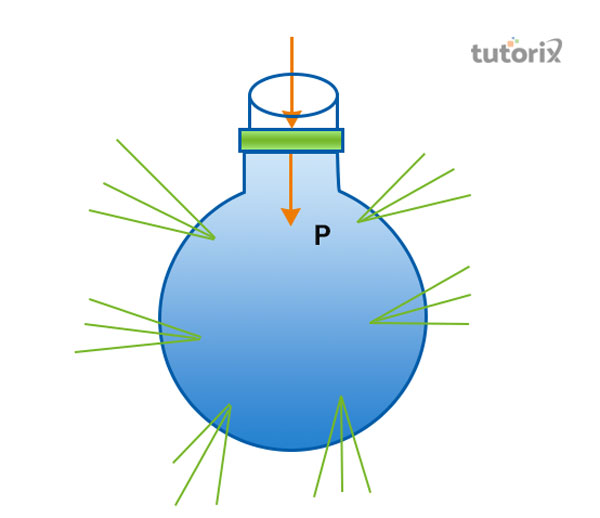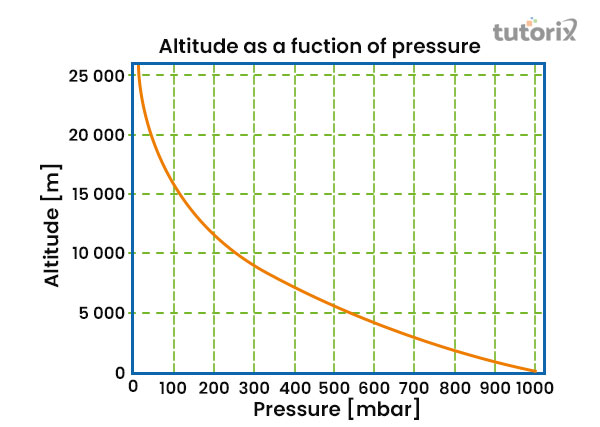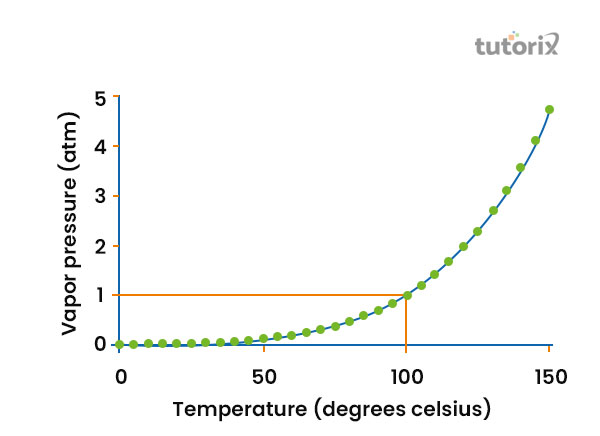# Relation Between Bar And Atm

## Introduction

Pressure is the term that is used in several sections of physics. The term, pressure is given in the form of different units.

The Bar and Atm are the two units that help in the representation of pressure.

The bar The Atm
as the pressure metric unit denote the atmospheric pressure

The word bar is derived from the Greek word, baros, which means weight.

## Relationship between bar and ATM

A bar is a metric unit that is used to determine the amount of pressure; however, this is not the part of the International System of Units, which uses SI units to determine the unit of measure any function.

The bar is used by the barometric formula; in this case, 1 bar roughly determines the atmospheric pressure, which is atm, being exerted on the planet at an altitude of 111 metres at 15 degree Celsius.

• The units of bar and millibar that are known, were introduced by Vilhelm Bjerknes, a Norwegian meteorologist.
• He is also known as the pioneer of modern weather forecasting practices. The bar is generally used to determine the atmospheric pressure in the countries of the European Union.
• The bar is therefore the metric unit of pressure and atm is determined as the standard atmospheric unit.
• The first denotes the amount of atmospheric pressure exerted on earth’s altitude of 111m at a temperature of 15 degrees Celsius (Efremov et al. 2021). On the other hand, Atm defines the average atmospheric pressure of earth at a sea level, one atm equal to 101, 325Pa or 101.325 kPa. The atmospheric pressure however is measured in millibars, the standard atmospheric pressure is equal to 1.01325 bar which equals to 101.325kPa.

Hence it can be established that 1 atm = 1.101325 barFigure 1: Pressure

## Bar

The term bar originated from the Greek word called baros, this term is associated with weight. Officially the symbol, bar that is used to be denoted by the alphabet b however currently it conflicts with the usage of this alphabet to denote the unit of barn. However, it can be encountered that the bars are now commonly denoted as mb rather than the property usage of mbar which denotes the millibars.

The standard atmospheric pressure is defined as the 101.325 kPa or 1013.25 mbar.

## Applications of bar

• The unit of the bar is utilized in various aspects. From metrology to oceanography the unit of the bar is used in various sections of measurements. The meteorologists and weather reporters for example measure the atmospheric pressure of air in millibars. In terms of oceanography, decibars are used to determine the unit of pressure on the sea level(ac, 2022).
• Aside from these sections of the study, bars are used in case of activities as well. In the case of scuba diving, the instructors mainly express the value of pressure in terms of bars. The unit is measured in bars in case of measurement of pressure in the tyres. Bars are also used in the field of vacuum engineering; the ‘residual pressure is the pressure of the remaining water supply of a particular flowing body of water that is expressed in millibars.Figure 2: Altitude and pressure in mbar

## Application of Atm

• The standard atmospheric pressure is Atm is primarily used as a reference unit for the measurement of the average atmospheric pressure at the sea level.
• Often it is used to determine the depth rating for water-resistant watches other than these aspects the atm is rarely used for measurement of the pressure units (Tadrist et al. 2019).
• It is also used in the aviation industry since the weather condition has its impact on the atmospheric pressure, the atmospheric pressure changes in the weather concerning altitude which calls for the need for standardisation of a single value to make a proper comparison of measurement and specifications. It is used to determine vapour pressure of liquid(purdue, 2022).
• Particularly, the value of 1 standard atmosphere is used by the aviation industry as a standardised measure to assess the sea-level pressure 1 standard atm equal to an exact value of 101,325 pascals or 101.325 kPa (Muller, Ervik & Mejía, 2020).Figure 3: Vapour pressure of liquid

## Conclusion

Bar and atm are the two common units of measurement for the pressure level present in the atmosphere. The bar is associated with the metric unit of pressure, however, it is not a part of the International system of units. The atm on the other hand is the standard unit of measurement for the atmospheric pressure which is equal to the value of 101325 pa. This unit is usually utilised to measure the average atmospheric pressure at the sea level of the earth.

## FAQs

Q1. What is the relation between Bar and Atm?

Ans. The key relation ‘between the units of bar and atm is that both of these are used to measure the pressure that is governed by the aspect of force per unit area. Mathematically, the relationship can be established in such a manner that 1 bar = .986923 atm and 1atm = 1.01325 bar.

Q2. What is 1 bar and 1 atm?

Ans. 1 atm is associated with the amount of pressure exerted by the column atmosphere that exists at the sea level and 1 bar resembles the unit of the pressure that is almost identical to the atmosphere Unit. For the measurement of both of these units, the fundamental unit is considered kg/ms2.

Q3. What is 1 atm as a standard pressure?

Ans. The standard atmospheric pressure is determined by Pa. Thus 1 atm of pressure value is equal to 101.325kpa.

Q4. In which field of application bar and atm are used?

Ans.The bar is used in the determination of the residual pressure of water for vacuum engineering. Atm is used by the aviation industry to determine the sea level pressure for proper calibration.

Q5. What is the value of standard atmospheric pressure?

Ans. The standard atmospheric pressure is 101.325 kpa. This is about 14.7 pounds of pressure per square inch.